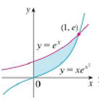Chapter 6.1, Problem 2E### Single Variable Calculus: Early Tr...

8th Edition
James Stewart
ISBN: 9781305270343

#### Solutions

Chapter
Section### Single Variable Calculus: Early Tr...

8th Edition
James Stewart
ISBN: 9781305270343
Textbook Problem

# Find the area of the shaded region.To determine

The area of the given shaded region.

Explanation

Given information:

The upper bound curve y=f(x) is 2x.

The lower bound curve y=g(x) is x24x.

The shaded region lies between x=0 and x=6. Hence, the limits are a=0 and b=6.

Calculation:

Calculate the area of the shaded region using the area formula.

A=abf(x)dxabg(x)dx (1)

Here, the area of region bounded by the upper curve is abf(x)dx and the area of region bounded by lower curve is abg(x)dx.

Substitute 2x for f(x), x24x for g(x), 0 for a, and 6 for b in Equation (1)

### Still sussing out bartleby?

Check out a sample textbook solution.

See a sample solution

#### The Solution to Your Study Problems

Bartleby provides explanations to thousands of textbook problems written by our experts, many with advanced degrees!

Get Started

#### In Exercises 58, evaluate the expression. 7. |2 6|

Applied Calculus for the Managerial, Life, and Social Sciences: A Brief Approach

#### 2. Define the terms population, sample, parameter and statistic.

Statistics for The Behavioral Sciences (MindTap Course List)

#### The range of is: (−∞,∞) [0, ∞) (0, ∞) [1, ∞]

Study Guide for Stewart's Multivariable Calculus, 8th

#### True or False: is a geometric series.

Study Guide for Stewart's Single Variable Calculus: Early Transcendentals, 8th